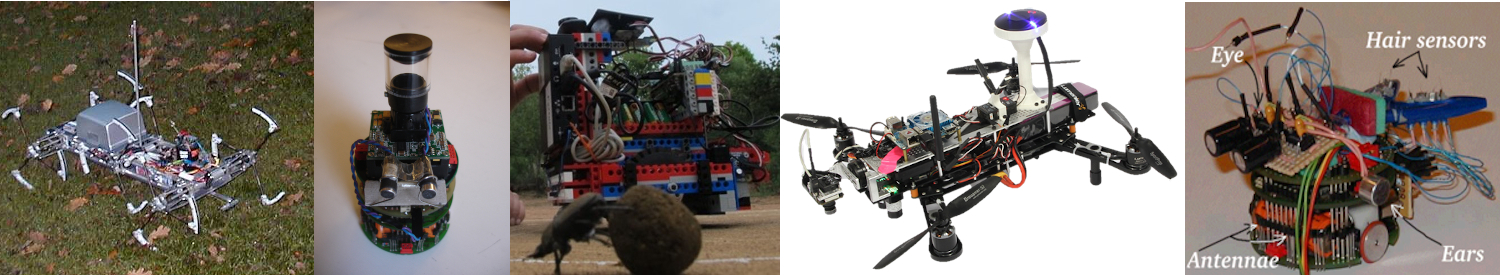# Insect Robotics Group

Building robots to understand insect behaviour

# AntBot: Tweaking the modules

The app should be in a working state, so now we shall do some very simple edits of the code.

To set this up properly, instantiate all 4 example modules as descrbied in the previous tutorial, call the Haferlach PI “HF” when registering it.

If you open up the WeightedCombiner you will see it works by taking the mean of all 4 modules, we’re going to edit this to give more preference to the Trig Path Integrator.

The combiner consists of a single function ‘nextCommand’ which works by calling the ‘getOpinion’ method on each module and storing their headings, it then performs some collection of operations on these to create it’s own heading to be returned.

In this case, the combiner simply takes the average of all 4 modules’ headings and returns this. Currently, the average gives an equal weighting to each – we want this to be skewed so jump to lines 28 and 29 and change the weighting of the modules from 0.25 on all to 0.7 on PI and 0.1 on the others.

``` double wAngle = PI * 0.7 + VH * 0.1 + SS * 0.1 + HF * 0.1; double wDist = PI * 0.7 + VH * 0.1 + SS * 0.1 + HF * 0.1; double[] weightedHeading = { wAngle, wDist }; ```

Now we have a weighted combiner that favours the trig PI. This is in essence the basics of how a combiner works – but what if we want to condition the weightings on the status of the modules?

More Tweaking the Combiner (Examining state of modules)

Each module has a method called ‘getStatus()’ which returns a string describing what it is doing. Looking at the source code for a module will tell you what the format of this string is.

In this case, we want to condition the weighting on the distance from the nest – as such we need to look at the status of the trig path integrator whose status is a string of the format <antbotOrientation> <angleFromNest> <distanceFromNest>.

We can retrieve and split this into tokens with

``` String[] PI_status = navigationModules.get("PI").getStatus().split("\\s+"); double nestDistance = Double.valueOf(PI_status); ```

Now we know the nest distance, we can simply surround the weighting with an if statement:

``` double wAngle; double wDist;```

if(nestDistance > 0.5)
{
wAngle = PI * 0.25 + VH * 0.25 + SS * 0.25 + HF * 0.25;
wDist = PI * 0.25 + VH * 0.25 + SS * 0.25 + HF * 0.25;
}
else
{
wAngle = PI * 0.7 + VH * 0.1 + SS * 0.1 + HF * 0.1;
wDist = PI * 0.7 + VH * 0.1 + SS * 0.1 + HF * 0.1;
}

Tweaking Visual Homing

To give a basic example of how tweaking a module would work, we shall change the type of pixelwise error calculation that the visual homing module uses.

Visual Homing works by taking in frames from the camera sensor  and calculating the average value of the pixels in the frame – this is done with the “calculateError()” function.

Open up VisualHoming and declare a new function “sumSquare()”:

```private double sumSquare() { // Get difference Core.subtract(currentFrame, homeFrame, diffFrame);```

``` // Get square Core.multiply(diffFrame, diffFrame, sqFrame); // Get sum of the array Scalar sum = Core.sumElems(sqFrame); double errorArr[] = sum.val; double ss = errorArr / 2; ```

```return ss; }```

Now go into calculateError() and replace “currentError = rootMeanSquare();” with “currentError = sumSquare();”.

Just like that we have modified the module.